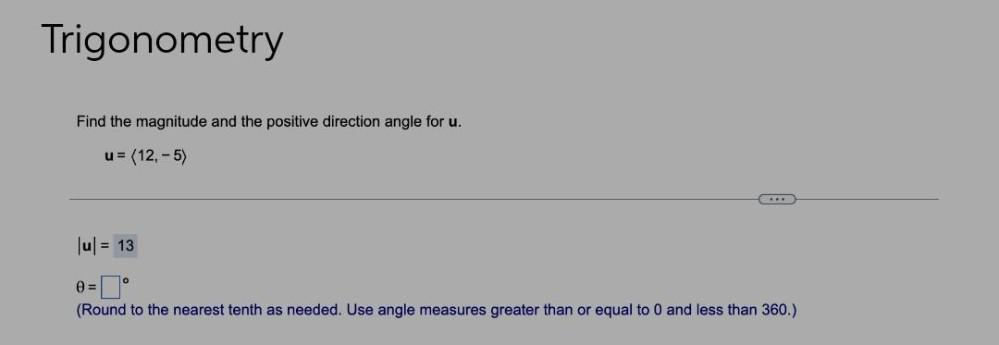Question:

# Find the magnitude and the positive direction angle for u. u = 〈12,-5〉 |u| = 13 ∅ =▢⁰ (Round to the nearest tenth as needed. Use angle measures greater than or equal to 0 and less than 360.)Find the magnitude and the positive direction angle for u. u = 〈12,-5〉 |u| = 13 ∅ =▢⁰ (Round to the nearest tenth as needed. Use angle measures greater than or equal to 0 and less than 360.)